# Introduction To Multicomponent Distillation Notes | Study Mass Transfer - Chemical Engineering

## Chemical Engineering: Introduction To Multicomponent Distillation Notes | Study Mass Transfer - Chemical Engineering

The document Introduction To Multicomponent Distillation Notes | Study Mass Transfer - Chemical Engineering is a part of the Chemical Engineering Course Mass Transfer.
All you need of Chemical Engineering at this link: Chemical Engineering

5.3. Introduction to Multicomponent Distillation
In industry, most of the distillation processes involve with more than two components. The multicomponent separations are carried out by using the same type of distillation columns, reboilers, condensers, heat exchangers and so on. However some fundamental differences are there which is to be thoroughly understood by the designer. These differences are from phase rule to specify the thermodynamic conditions of a stream at equilibrium. In multicomponent systems, the same degree of freedom is not achieved because of the presence of other components. Neither the distillate nor the bottoms composition is completely specified. The components that have their distillate and bottoms fractional recoveries specified are called key components. The most volatile of the keys is called the light key (LK) and the least volatile is called the heavy key (HK). The other components are called non-keys (NK). Light no-key (LNK) is referred when non-key is more volatile than the light key whereas heavy non-key (HNK) is less volatile than the heavy key. Proper selection of key components is important if a multicomponent separation is adequately specified. Several short-cut methods are used for carrying out calculations in multicomponent systems. These involve generally an estimation of the minimum number of trays, the estimation of minimum reflux rate and number of stages at finite reflux for simple fractionators. Although rigorous computer methods are available to solve multicomponent separation problems, approximate methods are used in practice. A widely used approximate method is commonly referred to as the Fenske-Underwood-Gilliland method.

5.3.1. Estimation of Minimum number of trays: Fenske Equation
Fenske (1932) was the first to derive an Equation to calculate minimum number of trays for multicomponent distillation at total reflux. The derivation was based on the assumptions that the stages are equilibrium stages. Consider a multicomponent distillation column operating at total reflux as shown in Figure 5.24.
Equilibrium relation for the light key component on the top tray is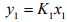(5.57)
For total condenser, y1 = xD then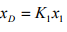(5.58)
An overall material balance below the top tray and around the top of the column can be written as: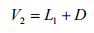(5.59)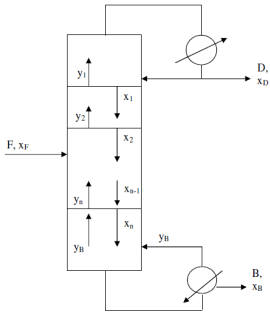Figure 5.24: Multicomponent column at minimum trays

Under total reflux condition, D = 0, thus, V2 = L1. The component material balance for the light key component around the first plate and the top of the column is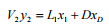(5.60)
Then under the conditions of the minimum trays, the Equation (5.60) yields y2 = x1. The equilibrium relation for plate 2 is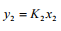(5.61)
The Equation (5.61) becomes at y2 = x1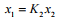(5.62)
Substituting Equation (5.62) into Equation (5.58) yields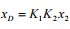(5.63)
Continuing this calculation for entire column, it can be written as: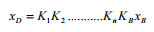(5.64)
In the same fashion, for the heavy key component, it can be written as: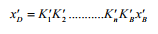(5.65)
Equation (5.64) upon Equation (5.65) gives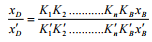(5.66)
The ratio of the K values is equal to the relative volatility, thus the Equation (5.66) can be written as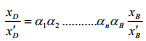(5.67)
If average value of the relative volatility applies for all trays and under condition of minimum trays, the Equation (5.67) can be written as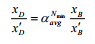(5.68)
Solving Equation (5.68) for minimum number of trays, Nmin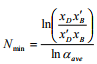(5.70)
The Equation (5.70) is a form of the Fenske Equation. In this Equation, Nmin is the number of equilibrium trays required at total reflux including the partial reboiler. An alternative form of the Fenske Equation can be easily derived for multi-component
calculations which can be written as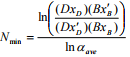(5.71)
The amount of light key recovered in the distillate is (DxD). This is equal to the fractional recovery of light key in the distillate say FRD times the amount of light key in the feed which can be expressed as: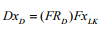(5.72)
From the definition of the fractional recovery one can write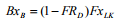(5.73)
Substituting Equations (5.72) and (5.73) and the corresponding Equations for heavy key into Equation (5.71) yields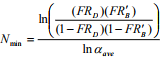(5.74)
Once the minimum number of theoretical trays, Nmin is known, the fractional recovery of the non-keys can be found by writing Equation (5.74) for a non-key component and either the light or heavy key. Then solve the Equation for FRNK,B or FRNK,D. If the key component is chosen as light key, then FRNK,D can be expressed as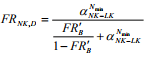(5.75)

4.3.2. Minimum Reflux: Underwood Equations
For multi-component systems, if one or more of the components appear in only one of the products, there occur separate pinch points in both the stripping and rectifying sections. In this case, Underwood developed an alternative analysis to find the minimum reflux ratio (Wankat, 1988). The presence of non-distributing heavy non-keys results a pinch point of constant composition at minimum reflux in the rectifying section whereas the presence of non-distributing light non-keys, a pinch point will occur in the stripping section. Let us consider the pinch point is in the rectifying section. The mass balance for component i around the top portion of the rectifying section as illustrated in
Figure 5.24 is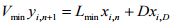(5.76)
The compositions are constant at the pinch point then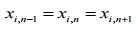(5.77)
and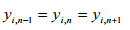(5.78)
The equilibrium relation can be written as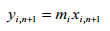(5.79)
From the Equations (5.76) to (5.79) a balance in the region of constant composition can be written as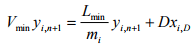(5.80)
Defining the relative volatility αi=mi/mHK and substituting in Equation (5.80) one can express after rearranging as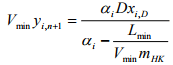(5.81)
The total vapor flow in the rectifying section at minimum reflux can be obtained by summing Equation (5.81) over all components as: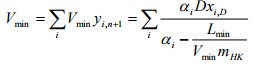(5.82)
Similarly after analysis for the stripping section, one can get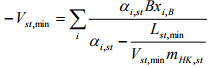(5.83)

Defining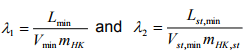(5.84)
Equations (5.82) and (5.83) then become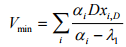(5.85)
and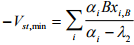(5.86)

For constant molar overflow and constant relative volatilities,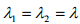that satisfies both Equations (5.86). The change in vapor flow at the feed stage ( ΔVF ) is then written as by adding the Equations (5.86)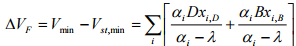(5.87)
Combining (5.87) with the overall column mass balance for component i can be expressed as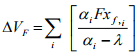(5.88)
Again if the fraction q is known, the change in vapor flow at the feed stage can be expressed as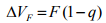(5.89)
Comparing Equation (5.88) and (5.89)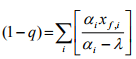(5.90)
Equation (5.90) is known as the first Underwood Equation which is used to calculate appropriate values of λ. whereas Equation (5.86) is known as the second Underwood Equation which is used to calculate Vm. From the mass balance Lm can be calculated as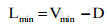(5.91)

4.3.3. Estimation of Numbers of Stages at Finite Reflux: Gilliland Correlation
Gilliland (1940) developed an empirical correlation to relate the number of stages N at a finite reflux ratio L/D to the minimum number of stages and to the minimum reflux ratio. Gilliland represented correlation graphically with (N - Nmin)/(N + 1) as y-axis and (R - Rmin)/(R + 1) as x-axis. Later Molokanov et al. (1972) represented the Gilliland correlation as: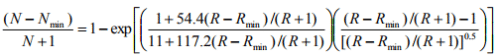(5.92)

According to Seader and Henley (1998), an approximate optimum feed-stage location, can be obtained by using the empirical Equation of Kirkbride (1944) as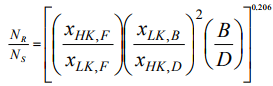(5.93)
where NR and NS are the number of stages in the rectifying and stripping sections, respectively.

Example problem 5.4:
A feed 100 kmoles/h of saturated liquid containing 10 mole % LNK, 55 mole % LK, and 35 mole % HK and is to be separated in a distillation column. The reflux ratio is 1.2 the minimum. It is desired to have 99.5 % recovery of the light key in the distillate. The mole fraction of the light key in the distillate should be 0.75. Equilibrium data: αLNK = 4.0, αLK = 1.0, αHK = 0.75.
Find
(i) Minimum number of stages required by Fenske method
(ii) Minimum reflux ratio by Underwood method
(iii) Number of ideal stages at R = 1.2 Rmin by Gilliland method
(iv) Also find the number of ideal stages at rectifying section and the stripping section at the operating reflux ratio and location of feed stage.

Solution 5.4:
(i) Feed F = 100 kmol/s, xLNK,F = 0.1, xLK,F = 0.55, xHK,F = 0.35, xLK,D = 0.75, FRLK,D = 0.995, aHK = 0.75, aLNK = 4.0, aLK = 1.0.
From the material balance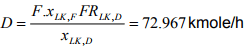Therefore W = F-D = 27.033 kmole/h
The amount of kmoles of different component in distillate:
nLND = F.xLNK,F.FRLK,D = 54.725 kmole/h
nLNK,D = F.xLNK,F = 10 kmole/h
nHK,D = D- nLK,D – nLNK,D = 8.242 kmole/h
The amount of kilo moles of different component in bottoms:
nLK,B = F. xLK,F (1- FRLK,D) = 0.275 kmole/h
nLNK,B = 0
nHK,B = B-nLK,B –nLNK,B = 26.758 kmole/h
a = 1/aHK
xHK,D = nHK,D/D = 0.113
xLK,B = nLK,B/B = 0.0102
Then as per Equation (5.71)
Nmin = 22.50

(ii) To find the minimum reflux at the condition of saturated liquid,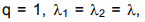using Equation (5.90)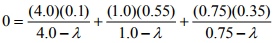gives λ= 0.83 Then from Equation (5.85)
Vmin = 253.5 kmole/h
And Lmin = Vmin – D = 180.53 kmole/h
Rmin = Lmin/D = 2.47

(iii) Now using the Gilliland correlation (Equation (5.92) to determine number of ideal stages at R = 1.2 Rmin =2.97 one can get N = 48.89

(iv) Using Kirkbride Equation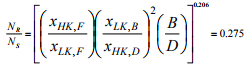Again NR + NS = N = 48.89
So by solving the above two Equations one get NR = 10.56 and NS = 38.34 and feed at stage 11

Nomenclature
B             Moles of bottoms
BPC        Bubble point curve
D            Moles of distillate
DPC       Dew point curve
Eo          Overall tray efficiency
Emv       Tray efficiency based on vapor phase
EmL       Tray efficiency based on liquid phase
F            Moles of feed
f             Molal fraction of feed
H           Enthalpy
K           Equilibrium constant
L           Moles of liquid
L ’         Moles of liquid in stripping section
N, n      Number of tray
P         Pressure, Pinch point
Q         Rate of heat transfer
R         Reflux ratio
V         Moles of vapor
V ’       Moles of vapor in stripping section
x         mole fractions in liquid
y         mole fractions in vapor
T        Temperature
α         Relative volatility
µ         Viscosity

Subscripts
B         Bottom
C         Condenser
D         Distillate
F         Feed
HK     Heavy key
i         1, 2, 3, …., n
L         Liquid
LK       Light key
min      Minimum
NK       Non key
R         Rectifying section
S         Stripping section
V         Vapor

The document Introduction To Multicomponent Distillation Notes | Study Mass Transfer - Chemical Engineering is a part of the Chemical Engineering Course Mass Transfer.
All you need of Chemical Engineering at this link: Chemical EngineeringUse Code STAYHOME200 and get INR 200 additional OFF

## Mass Transfer

29 videos|45 docs|44 tests

Track your progress, build streaks, highlight & save important lessons and more!

,

,

,

,

,

,

,

,

,

,

,

,

,

,

,

,

,

,

,

,

,

;